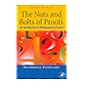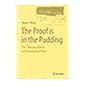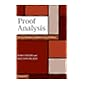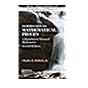Normal view MARC view ISBD view

Introduction to mathematical proofs: a transition to advanced Mathematics

Material type:BookSeries: Textbooks in mathematics. Publisher: Boca Raton CRC Press 2015Edition: 2nd ed.Description: xiv, 400 p.ISBN: 9781482246872.DDC classification: 511.36 Summary: Introduction to Mathematical Proofs helps students develop the necessary skills to write clear, correct, and concise proofs. Unlike similar textbooks, this one begins with logic since it is the underlying language of mathematics and the basis of reasoned arguments. The text then discusses deductive mathematical systems and the systems of natural numbers, integers, rational numbers, and real numbers. It also covers elementary topics in set theory, explores various properties of relations and functions, and proves several theorems using induction. The final chapters introduce the concept of cardinalities of sets and the concepts and proofs of real analysis and group theory. In the appendix, the author includes some basic guidelines to follow when writing proofs. This new edition includes more than 125 new exercises in sections titled More Challenging Exercises. Also, numerous examples illustrate in detail how to write proofs and show how to solve problems. These examples can serve as models for students to emulate when solving exercises. Several biographical sketches and historical comments have been included to enrich and enliven the text. Written in a conversational style, yet maintaining the proper level of mathematical rigor, this accessible book teaches students to reason logically, read proofs critically, and write valid mathematical proofs. It prepares them to succeed in more advanced mathematics courses, such as abstract algebra and analysis. (https://www.crcpress.com/Introduction-to-Mathematical-Proofs-Second-Edition/Roberts/9781482246872)
Tags from this library: No tags from this library for this title.
average rating: 0.0 (0 votes)
Item type Current location Item location Collection Call number Status Date due Barcode
Books Vikram Sarabhai Library
Slot 1355 (0 Floor, East Wing) Non-fiction 511.36 R6I6 (Browse shelf) Available 190939
Browsing Vikram Sarabhai Library Shelves , Collection code: Non-fiction Close shelf browser511.36 C8N8-2013 The nuts and bolts of proofs: an introduction to mathematical proofs 511.36 K7P7 The proof is in the pudding: the changing nature of mathematical proof 511.36 N3P7 Proof analysis: a contribution to Hilbert's last problem 511.36 R6I6 Introduction to mathematical proofs: a transition to advanced Mathematics

1. Logic

Statements, Negation, and Compound Statements
Truth Tables and Logical Equivalences
Conditional and Biconditional Statements
Logical Arguments
Open Statements and Quantifiers
Chapter Review

2. Deductive Mathematical Systems and Proofs

Deductive Mathematical Systems
Mathematical Proofs
Chapter Review

3. Set Theory

Sets and Subsets
Set Operations
Generalized Set Union and Intersection
Chapter Review

4. Relations

Relations
The Order Relations <, , >,
Reflexive, Symmetric, Transitive, and Equivalence Relations
Equivalence Relations, Equivalence Classes, and Partitions
Chapter Review

5. Functions

Functions
Onto Functions, One-to-One Functions and One-to-One Correspondences
Inverse of a Function
Images and Inverse Images of Sets
Chapter Review

6. Mathematical Induction

Mathematical Induction
The Well-Ordering Principle and the Fundamental Theorem of Arithmetic

7. Cardinalities of Sets

Finite Sets
Denumerable and Countable Sets
Uncountable Sets

8. Proofs from Real Analysis

Sequences
Limit Theorems for Sequences
Monotone Sequences and Subsequences
Cauchy Sequences

9. Proofs from Group Theory

Binary Operations and Algebraic Structures
Groups
Subgroups and Cyclic Groups

Appendix Reading and Writing Mathematical Proofs

Answers to Selected Exercises

References

Index

Introduction to Mathematical Proofs helps students develop the necessary skills to write clear, correct, and concise proofs.

Unlike similar textbooks, this one begins with logic since it is the underlying language of mathematics and the basis of reasoned arguments. The text then discusses deductive mathematical systems and the systems of natural numbers, integers, rational numbers, and real numbers.

It also covers elementary topics in set theory, explores various properties of relations and functions, and proves several theorems using induction. The final chapters introduce the concept of cardinalities of sets and the concepts and proofs of real analysis and group theory. In the appendix, the author includes some basic guidelines to follow when writing proofs.

This new edition includes more than 125 new exercises in sections titled More Challenging Exercises. Also, numerous examples illustrate in detail how to write proofs and show how to solve problems. These examples can serve as models for students to emulate when solving exercises.

Several biographical sketches and historical comments have been included to enrich and enliven the text. Written in a conversational style, yet maintaining the proper level of mathematical rigor, this accessible book teaches students to reason logically, read proofs critically, and write valid mathematical proofs. It prepares them to succeed in more advanced mathematics courses, such as abstract algebra and analysis.

(https://www.crcpress.com/Introduction-to-Mathematical-Proofs-Second-Edition/Roberts/9781482246872)

There are no comments for this item.